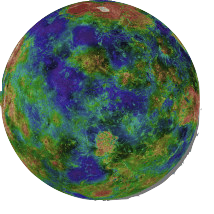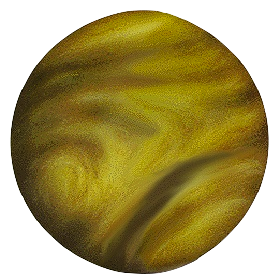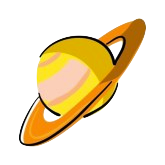• Uniform
• Normal
• Exponential
• Lognormal

# Statistical Inference

For the next few weeks, we're going to be studying techniques for “statistical inference.” The central question of inference is, “What are we entitled to say about the real world based on the data we have collected?” The techniques involve a pattern of logic that is counter-intuitive to many people. It involves conditional reasoning — thinking about what would happen if certain assumptions (called “hypotheses”) were true.

It's easy to forget that the reasoning is conditional, that assuming a hypothesis to be true does not make it true, and for this reason there are many misconceptions about statistical inference.

We'll start with a more intuitive approach to inference, called the Bayesian approach. The Bayesian approach is itself a worthy object of study and provides a powerful set of techniques for reasoning about the world. However, the natural and social sciences are only slowly moving toward the use of the Bayesian approach. Almost all technical literature is written using the Frequentist perspective. For that reason, we won't be using the Bayesian approach directly in this course. Nevertheless, people seem to achieve a better grasp on the frequentist approach to hypothesis testing if they are able to contrast it with the Bayesian approach; it seems to be easier to overcome your intuition if you understand what your intuition is telling you.

### Conditional Probabilities

We've talked about probabilities. Now, we're going to be explicit about the setting in which a probability applies, called the condition.

In statistical evidence, we want to draw a conclusion about the world based on some data. A nice form for such conclusions is as a probability: the probability of the world being in a particular state given the data: p(world | data)

But our knowledge of the world often takes a different form: p( data | world ) — if the world is a particular way, what are we likely to see. Example: the Higgs Boson and energies of 125 TeV. Make an assumption about the world: e.g. the standand model of physics and a corresponding energy for the Higgs Boson.

But what we are really interested in is p( world | data )
Look at the data from the Large Hadron Collider at CERN. How do we convert this into a statement about the world.

Example: Suppose that you are interested in using headaches to diagnose whether someone has a flu or not. Based on surveys of lots of people, you come up with the following data:

Condition Flu Healthy

These are conditional probabilities: probabilities conditioned on whether or not a person has the flu. The are often written using notation like this: prob(Headache|Flu)

Such conditional probabilities are often based on direct evidence; they are given.

Now the problem. You have a headache. What's the probability that you have the flu?

• Note that the column totals are 1.00. The row totals don't have any obvious meaning.
• Introduce prior probability; the probability that you have the flu before you consider the data.
• Let's set the prior for flu to be p(flu) = 0.02, and therefore the probability of being healthy is 0.98.

Here's the basic rule for combining prior and conditional probabilities to construct a joint probability: p(flu & headache) = p(headache|flu) p(flu)

The tabular approach: construct joint probabilities.

The tree approach: construct all the branches of the tree and then cross out those that aren't consistent with the evidence.

EXAMPLE: Mammography

Sensitivity: 90% probability of a positive test if the woman has breast cancer

Specificity: 90% probability of a negative test if the woman does not have breast cancer.

Prevalence: 1%

You have a positive result. What's the probability that you have breast cancer?

# The Prior

Calculating a posterior probability requires a prior.

### What to do when you have no prior?

Simply assert that you are in the world of one hypothesis or another and see what happens in that world.

# The Multi-World Metaphor for Statistical Inference

Motto: Always know what world you are thinking about.

Statistical inference is hard to learn. It will help if you can understand the underlying logic. This will help you avoid major mis-interpretation of the results of statistical inference techniques.For example, even among professional scientists, the mainstream (mis)-understanding of p-values is that they reflect the probability that the null hypothesis is correct.

Part of the reason why statistical inference is hard to grasp is that the logic is genuinely hard. It involves contrapositives, it involves conditional probabilities, it involves “hypotheses.'' And what is a hypothesis? To a scientist, it is a kind of theory, an idea of how things work, an idea to be proven through lab or field research or the collection of data.

But statistics hews not to the scientist's but to the philosopher's or logician's rather abstract notion of a hypothesis: a proposition made as a basis for reasoning, without any assumption of its truth.'' (Oxford American Dictionaries) Or more simply:

"A hypothesis is a statement that may be true or false.”

What kind of scientist would frame a hypothesis without some disposition to think it might be true? Only a philosopher or a logician.

To help connect the philosophy and logic of statistical hypotheses with the sensibilities of scientists, it might be helpful to draw from the theory of kinesthetic learning — an active theory, not a philosophical proposition — that learning is enhanced by carrying out a physical activity. Many practitioners of the reform style of teaching statistics engage in the kinesthetic style; they have students draw M&Ms from a bag and count the brown ones, they have students walk randomly to see how far they get after $$n$$ steps, or, as we do in this class, they toss a globe around the classroom to see how often a students' index finger lands in the ocean.

To teach hypothesis testing in a kinesthetic way, you need a physical representation of the various hypotheses found in statistical inference. One way to do this involves not the actual physical activity of truly kinesthetic learning, but concrete stories of making different sorts of trips to different sorts of worlds.A hypothesis may be true on some worlds and false on others. On some planets we will know whether a hypothesis is true or false, on others, the truth of a hypothesis is an open question.

Of course, the planet that we care about, the place about which we want to be able to draw conclusions. It's Planet Earth:We want to know which hypotheses are true on Earth and which are false.

But Earth is a big and complicated place; it's hard to know exactly what's going on. So, to deal with the limits of our abilities to collect data and to understand complexity, statistical inference involves a different set of planets. These planets resemble Planet Earth in some ways and not in others. But they are simple enough that we know exactly what's happening on them, so we know which hypotheses are true and which are false on these planets.

These planets are:

Planet Sample Planet Null Planet AltPlanet Sample is populated entirely with the cases we have collected on Planet Earth. As such, it somewhat resembles Earth, but many of the details are missing, perhaps even whole countries or continents or seas. And of course, it is much smaller than Planet Earth.

Planet Null is a boring planet. Nothing is happening there. Express it how you will: All groups all have the same mean values. The model coefficients are zero. Different variables are unrelated to one another. Dullsville. But even if it's dull, it's not a polished billiard ball that looks the same from every perspective. It's the clay from which Adam was created, the ashes and dust to which man returns. But it varies from place to place, and that variation is not informative. It's just random.

Finally, there is Planet Alt. This is a cartoon planet, a caricature, a simplified representation of our idea of what is (or might be) going on, our theory of how the world might be working. It's not going to be exactly the same as Planet Earth. For one thing, our theory might be wrong. But also, no theory is going to capture all the detail and complexity of the real world.

In teaching statistical inference in a pseudo-kinesthetic way, we use the computer to let students construct each of the planets. Then, working on that planet, the student can carry out simulations of familiar statistical operations. Those simulations tell the student what they are likely to see if they were on that planet. Our goal is to figure out whether Earth looks more like Planet Null or more like Planet Alt.

For the professional statisticians, the planet metaphor is unnecessary. The professional has learned to keep straight the various roles of the null and alternative hypothesis, and why one uses a sample standard error to estimate the standard deviation of the sampling distribution from the population. But most students find the array of concepts confusing and make basic categorical errors. The concrete nature of the planets simplifies the task of keeping the concepts in order. For instance, Type I errors only occur on Planet Null. You have to be on Planet Alt to make a Type II error. And, of course, no matter how many (re)samples we make on Planet Sample, it's never going to look more like Earth than the sample itself.